# SAS/ETS Examples

## Multiple Imputation for a GARCH(1,1) Model

Contents | SAS Program

# OverviewFigure 1: Comparison of Density Estimates for the ARCH 1 Parameter Estimator Under Different Imputation Methods

Missing data in time series constitutes a problem that is not easily solved. Standard techniques available for cross-sectional data may not be applicable due to the dynamic nature of the models. Ad hoc procedures may either ignore some important facts or be difficult to implement.

Multiple imputation has been used for several years with cross sectional models to deal with missing data. Its flexibility makes it an adequate tool for times series data as well.

In this example, you will see how to implement a simple form of multiple imputation for time series to fit a GARCH(1,1) model when some of the data are missing. The only tools that you will need are the MODEL procedure, the MIANALYZE procedure, and some DATA Step statements.

# Details

Missing observations in times series data can be a difficult problem to overcome. Due to the dynamic nature of the models, simple techniques in use for cross-sectional models, like row wise deletion, are not applicable or may lead to questionable results.

One common way to deal with it is to employ a Kalman filter (Kohn and Ansley 1986). However, this usually requires the additional task of rewriting the model in state-space form, which may be difficult or may not be supported by the currently available software, if, for example, the model is nonlinear, like a GARCH model.

Single imputation (SI) is another common way to deal with missing data. Each missing value is replaced by an imputed value, for example by interpolation. The replaced values are then treated as if they were actual data values. This approach enables analysis with procedures designed for complete data sets. While this method is simple and can be applied to virtually every data set, it does not account for the uncertainty about the predictions of the imputed values. Therefore, the estimated variances of the parameters are biased toward zero, leading to statistically invalid inferences, in the sense of Rubin (1987).

Multiple Imputation (MI) is a methodology for dealing with incomplete data that has received considerable attention in the last couple of decades (see, for example, Rubin 1987 and 1996, and Schafer 1997.) It maintains the flexibility and relative ease of application of single imputation, while taking into account the variability due to the imputation of the missing values. Instead of filling each missing value with only one single value, MI imputes a set of m plausible values that represent the uncertainty about the right value. This approach creates m imputed replications of the original data sets that can be analyzed by standard procedures for complete data sets.

If Q is the quantity of interest, then the analysis of the m imputed data sets will produce m estimates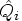of Q, with the corresponding estimates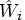of their variance.

The overall estimateof Q is the average of the m complete-data estimates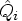:
As shown by Rubin (1987), the variance V of the multiple imputation point estimate is the average of the estimated variances within each complete data set,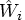, plus the sample variance of the point estimates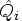between the datasets, multiplied by a correction factor.
whereis the sample variance of the estimatedbetween the datasets.

You can refer to "The MI Procedure" and "The MIANALYZE Procedure" chapters in the SAS/STAT User's Guide for more details on multiple imputation.

The multiple imputation literature so far has focused mainly on applications in cross-sectional models for survey data. Only recently, panel and time series data have been considered (see King et al. 2001, and Hopke, Chuanhai, and Rubin 2001.) In the same fashion, software for multiple imputation, like the MI procedure, supports mainly cross-sectional models. Although, from a theoretical point of view, there is no reason not to use multiple imputation for time series data, its application has been difficult in practice.

In the following example you will see how to use the Monte Carlo feature within the MODEL procedure to implement a simple form of multiple imputation, and to estimate a zero mean GARCH(1,1) model with missing data. Other algorithms of multiple imputation (see Hopke, Chuanhai, and Rubin 2001) may be more appropriate, however, this method is of very simple implementation, and provides results that are statistically superior to simple imputation in the case that is presented.

The MIANALYZE procedure can then combine the results from the different imputed data. The procedure has been designed to provide for great flexibility and enables you to analyze output obtained using a BY statement from virtually any SAS procedure. It reads parameter estimates and associated standard errors, and derives valid inferences according to the preceding formulas.

The GARCH(1,1) model with mean zero is described by the following equations:
The parameter vector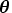is given by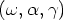.

For this example, a total of 1,000 data points are simulated from the above model, where the true values of the parameters are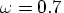,, and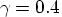. Then, about 10% of the original data are randomly set to missing. (The full code can be found in the accompanying SAS source file .)

The estimation procedure can be divided into four main steps.

1. The first step is to find some plausible preliminary estimates of the model parameters. One way to accomplish this is to use single imputation by replacing the missing observation with the mean, which, in this case, is zero. Other possible options include single imputation with polynomial interpolation, or using the longest set of data that does not contain missing observations.

   data single;
set missing;
if y=. then y=0; /* replace y with mean */
run;


The following PROC MODEL statements estimate the parameters of the GARCH(1,1) model using the dataset in which the missing data are replaced by the expected value. The estimated parameter vector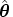is displayed in Figure 2.

   proc model data=single;
parms arch0 arch1 garch1;
/* Mean zero model --------*/
y = 0;
/* Garch Variance ---------*/
h.y = arch0 + arch1 * xlag( resid.y**2, mse.y) +
garch1 * xlag( h.y, mse.y ) ;
/* Fit the garch model ----*/
fit y   / data=single method=marquardt fiml outest=prelest
outcov outs=s prl=both;
bounds arch0 arch1 garch1 >= 0;
title 'Single imputation';
run;


 Single imputation

The MODEL Procedure

Nonlinear FIML Parameter Estimates
Parameter Estimate Approx Std Err t Value Approx
Pr > |t|
arch0 0.60608 0.1619 3.74 0.0002
arch1 0.24358 0.0458 5.31 <.0001
garch1 0.472489 0.1003 4.71 <.0001

Parameter Wald
95% Confidence Intervals
Parameter Value Lower Upper
arch0 0.6061 0.2888 0.9233
arch1 0.2436 0.1537 0.3334
garch1 0.4725 0.2759 0.6691

Parameter Likelihood Ratio
95% Confidence Intervals
Parameter Value Lower Upper
arch0 0.6061 0.4855 0.9233
arch1 0.2436 0.2094 0.3334
garch1 0.4725 0.3978 0.5472

Figure 2: Single Imputation Estimates

The estimate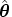, and its estimated covariance matrix, are saved in the prelest data set. Likewise, the estimated standard error of the residual is saved in the s data set.

2. The second step is to generate the imputed data. First define how many you want. In this case, 20 sets of imputations are requested.

    %let m=20; /* number of imputations */


Then generate them with the Monte Carlo feature within the MODEL procedure. The following SOLVE statement creates m replications of the input data with missing values replaced by imputed data. It accomplishes this by drawing m vectors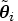of parameters from a multivariate normal distribution whose mean and covariance matrix are the estimated parameter vectorand covariance matrix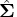. Bollerslev (1986) proved that the asymptotic distribution of the maximum likelihood estimators of a GARCH model is multivariate normal. Therefore, this choice of distribution seems appropriate.

The errors et are simulated from a univariate normal distribution with mean zero, while the standard error is taken from the s data set. The SOLVE statement supports simulation from several other distributions. Refer to the "Details: Simulation" section of "The Model Procedure" chapter in the SAS/ETS User's Guide for more details.

      /* Impute the missing point in DATA=missing data set */
solve y / data=missing estdata=prelest sdata=s
random=&m seed=123 out=monte forecast;


The 20 sets of imputed data values are saved in the monte output data set and are identified by the _REP_ variable. The data corresponding to _REP_=0 are the original data values.

The following output in Figure 3 shows that the last two missing values on the original data set are replaced by imputed values in the first two imputed replications of the data set, while the rest of the nonmissing data is kept unchanged. That is accomplished by the FORECAST option of the SOLVE statement.

 Original data set

Obs y
989 2.18025
990 -1.97288
991 .
992 -1.29694
993 -1.48274

 First set of imputed data

Obs _REP_ y
1989 1 2.18025
1990 1 -1.97288
1991 1 -3.74653
1992 1 -1.29694
1993 1 -1.48274

 Second set of imputed data

Obs _REP_ y
2989 2 2.18025
2990 2 -1.97288
2991 2 -2.94843
2992 2 -1.29694
2993 2 -1.48274

Figure 3: Multiply Imputed Data Sets

3. The next step is to reestimate the model for each set of imputed values using the BY statement. The starting values of the parameters are the estimates of the single imputation. The original set of data, corresponding to _REP_=0, is dropped from the input data set.

It is important to note that the estimated model is the same as the one used for imputation. In the words of Shafer (1997), the imputer's model coincides with the analyst's model, so that any problem arising from a discrepancy is avoided. Refer to "The MI Procedure" chapter in the SAS/STAT User's Guide and Schafer (1997) for an in-depth discussion about the choice of the imputer's model versus the analyst's model.

      /* Fit again y with the imputed data */
fit y   / data=monte(where=(_rep_>0)) method=marquardt fiml
estdata=prelest sdata=s nools outest=imp_est outcov;
bounds arch0 arch1 garch1 >= 0;
by _rep_;
title 'Multiple Imputation';
run;


The NOOLS option skips the preliminary OLS estimation that PROC MODEL does by default to find starting values for the parameters, since here the starting values can be provided by the single imputation estimates.

4. The last step is to summarize the results with the MIANALYZE procedure. The OUTEST= data set of PROC MODEL needs to be converted into a type=EST data set, and the name of the BY variable needs to be _Imputation_ in order to be processed by MIANALYZE.

   data mi_in_est(type=EST);
set imp_est;
rename _rep_ = _Imputation_;
if _name_="" then _type_="PARMS";
else _type_="COV";
run;


The following MIANALYZE statements produce parameter estimates and test statistics for the parameters of interest. The relevant part of the output is shown in Figure 4. The EDF= option specifies the complete-data degrees of freedom. Refer to "The MIANALYZE Procedure" chapter in the SAS/STAT User's Guide for more details on this option.

   proc mianalyze data=mi_in_est edf=1000;
modeleffects arch0 arch1 garch1;
run;


The MIANALYZE Procedure

Multiple Imputation Parameter Estimates
Parameter Estimate Std Error Std Error 95% Confidence Limits DF
arch0 0.680916 0.198299 0.198299 0.289496 1.072336 171.59
arch1 0.301525 0.061156 0.061156 0.181066 0.421983 245.08
garch1 0.422048 0.117238 0.117238 0.190272 0.653823 140.69

Figure 4: Multiple Imputation Parameter Estimates

To verify that this method produces results that are statistically superior to single imputation, a Monte Carlo experiment was conducted.

In this experiment, 10,000 time series were simulated from a GARCH(1,1) model, and the nominal 95% confidence intervals were computed both in the case of single imputation where the missing values were replaced either by the expected value or by polynomial interpolation, and in the case of multiple imputation according to the scheme outlined above.

The results are shown in Figure 5. The type variable indicates the type of confidence intervals: Wald for the complete data ('wald_full'), likelihood ratio for the complete data ('likl_full'), Wald computed with single imputation by mean replacement ('wald_mean'), likelihood ratio computed with single imputation by mean replacement ('likl_mean'), Wald computed with single imputation by interpolation ('wald_inte'), likelihood ratio computed with single imputation by interpolation ('likl_inte'), or with multiple imputation ('mi').

The actual coverage of the confidence intervals obtained with multiple imputation is much closer to the nominal coverage than the one of confidence intervals obtained with single imputation. Therefore, multiple imputation according the the procedure just outlined leads to better statistical inference, in the sense of Rubin (1987), for this particular model.

Figure 1 shows also that the distribution of mi estimates of the arch1 parameter is closer in shape and location to the distribution of the estimates when the complete data set is used.

Obs type coverage
1 wald_full 0.9406
2 likl_full 0.7236
3 mi 0.9445
4 wald_mean 0.8501
5 likl_mean 0.7245
6 wald_inte 0.7458
7 likl_inte 0.5687

Obs type coverage
8 wald_full 0.9452
9 likl_full 0.7512
10 mi 0.9136
11 wald_mean 0.7652
12 likl_mean 0.7186
13 wald_inte 0.7412
14 likl_inte 0.6423

Obs type coverage
15 wald_full 0.9359
16 likl_full 0.8173
17 mi 0.9409
18 wald_mean 0.8616
19 likl_mean 0.7789
20 wald_inte 0.7554
21 likl_inte 0.6635

Figure 5: Actual Coverage of 95% Confidence Intervals

The source code for the Monte Carlo study is available upon request. The %Distribute macro system of Doninger and Tobias (2001) has provided the tools to perform the study within a reasonable amount of time.

# References

Bollerslev, T. (1986), "Generalized Autoregressive Conditional Heteroskedasticity," Journal of Econometrics, 31, 307--327.

Doninger, C. and Tobias, R. (2001), "The %Distribute System for Large-Scale Parallel Computation in the SAS System." [http://www.sas.com/rnd/app/papers/distConnect.pdf] . Cary, NC: SAS Institute, Inc. Accessed 12 October 2001.

Hopke, P. K., Chuanhai, L., and Rubin, D. B. (2001), "Multiple Imputation for Multivariate Data with Missing And Below-Treshold Measurement: Time-Series Concentration of Pollutants in the Artic," Biometrics, 57, 22--33.

King, G., Honaker, J., Joseph, A., and Scheve, K. (2001), "Analyzing Incomplete Political Science Data: An Alternative Algorithm for Multiple Imputation," American Political Science Review, 95, 49--69.

Kohn, R. and Ansley, C.F (1986), "Efficient Estimation and Prediction in Time Series Regression Models," Biometrica, 72, 694--697.

Rubin, D.B. (1987), Multiple Imputation for Nonresponse in Surveys, New York: John Wiley & Sons, Inc.

Rubin, D. (1996), "Multiple Imputation after 18+ Years," Journal of the American Statistical Association, 91, 473--489.

Schafer, J.L. (1997), Analysis of Incomplete Data, London: Chapman and Hall.

SAS Institute, Inc. (2000), SAS/ETS User's Guide, Version 8.2, Cary, NC: SAS Institute, Inc.

SAS Institute, Inc. (2000), SAS/STAT User's Guide, Version 8.2, Cary, NC: SAS Institute, Inc.